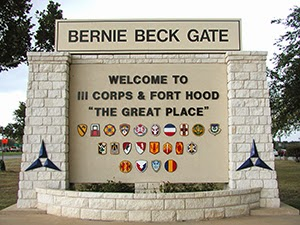## Wednesday, April 2, 2014

### April Fools | Fort Hood Shooting 2014 and 2009 | Name Numerology TellsBernie Beck Gate has quite the numerology...
Get a load of the numerology on Bernie Beck Gate, the entry to Fort Hood in Killeen, Texas.  It is a good starting point for what will follow in this post.
• Bernie = 2+5+18+14+9+5 = 53
• Beck = 2+5+3+11 = 21
• Bernie Beck = 53+21 = 74
• Gate = 7+1+20+5 = 33
• Bernie Beck Gate = 74 33
• Jesus was crucified at 33
• Jesus = 10+5+19+21+19 = 74
• Cross = 3+18+15+19+19 = 74
On November 5, 2009, Nidal Malik Hasan, shot and killed 13 people and injured 32 in a shooting spree at Fort Hood army base, at least so the story goes.
• Nidal = 14+9+4+1+12 = 40
• Malik = 4+1+12+9+11 = 37
• Hasan = 8+1+19+1+14 = 43
• Nidal Malik Hasan = 40+37+43 = 120
Notice how each of the three names have a 411 coded into it.  Also notice how the middle and last name have a coded 911 in them.  Now let us decode the names with the Pythagorean method, in contract with the standard method above.
• Nidal = 5+9+4+1+3 = 22
• Malik = 4+1+3+9+11 = 28
• Hasan = 8+1+1+1+5 = 16
• Nidal Malik Hasan = 22+28+16 = 66
Isn't it interesting?  It is the number 66, which is the numerology of the word 'freedom'.
• Freedom = 6+18+5+5+4+15+13 = 66
Today, April 2, 2014, another mass shooting occurred at the same Fort Hood military base located in Texas.
• Fort = 6+6+9+2 = 23
• Hood = 8+6+6+4 = 24
• Fort Hood = 23+24 = 47
Also, in Standard Method
• Fort = 6+15+18+20 = 59
• Hood = 8+15+15+4 = 42
• Fort Hood = 59+42 = 101
Today, the killer at Fort Hood is named Ivan Lopez; it is reported he killed three people before himself, bringing the death toll to a total of 4.  Also 16 additional people were injured.  The shooting took place in Bell County and the spokesperson for the shooting has been Mark A. Milley.
• Bell = 2+5+12+12 = 31
• Mark = 13+1+18+11 = 43
• Milley = 13+9+12+12+5+25 = 76
• Mark Milley = 43+76  = 119
Then there is Ivan Lopez.  In decoding this name, we will use the K/V exception on Ivan, where all letters are reduced with the Pythagorean method except K and V because they are masters numbers, 11 and 22 respectively.
• Ivan = 9+22+1+5 = 37
• Lopez = 3+6+7+5+8 = 29
• Ivan Lopez = 37+29 = 66
The name can also be decoded with the standard method.
• Ivan = 9+22+1+14 = 46
• Lopez = 12+15+16+5+26 = 74
• Ivan Lopez = 46+74 = 120
Isn't it strange, both shooters have numerology of 66 for names when decoded with Gematria.  Ivan Lopez is reported to be 34 years old, like the Georgia Guidestones on the 34th Parallel North, and a 4-month veteran of the Iraq War before returning to the U.S. from combat due to 'brain trauma'.  At this time, the mainstream media is reporting that Ivan Lopez had mental illness.

Fort Hood is located in Killeen, Texas
• Killeen = 11+9+12+12+5+5+14 = 68
• Texas = 20+5+24+1+19 = 69
And with the Pythagorean method.
• Killeen = 2+9+3+3+5+5+5 = 32
• Texas = 2+5+6+1+1 = 15
• Killeen, Texas = 32+15 = 47
With numerology like Killeen, Texas, there is no doubt that it is ripe for false flags...